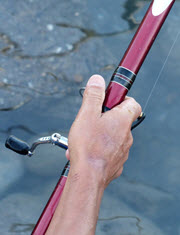# Moment or Torque

Moment, or torque, is a turning force.Moment: Force times the Distance at right angles.You can feel moment when holding onto a fishing rod.

As well as holding up its weight you have to stop it from rotating downwards.

### Example: 120 Newtons of force at 0.3 mM = 120 N × 0.3 m = 36 Nm## At Right Angles

Remember to use the distance at right angles to the force.

A different angle and we get less moment.

And pushing directly towards the bolt produces no moment at all.

### Example (continued): 115° instead of 90°M = 120 N × 0.3 m × sin(115°) = 32.63... Nm

## Levers### Example: What is the Force F?

The moment from the 500 N is:

500 N × 0.7 m = 350 N m

That will equal the moment from force F:

F × 0.07 m = 350 N m

Rearrange:

F = 350 N m / 0.07 m = 5000 N

It is 10 times larger.

Levers are so important that there are names for each part: Effort, Load and Fulcrum:## Moment on a Beam

This beam is stuck into the wall (called a cantilever):The Free Body Diagram looks like this:The upwards force R balances the downwards Weight.

With only those two forces the beam will spin like a propeller!

But there is also a Moment that balances it out.

### Example: Our guy has a mass of 80 kg, causing 785 N of force

785 N acting 3.2 m from the wall causes a moment of:

M = 785 N × 3.2 m = 2512 Nm

And that moment is what stops the beam from rotating. (Imagine the wall was poorly made, what would happen when our guy stands on the beam?)